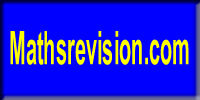# Higher

The Higher Course in Mathematics develops learners’ mathematical rigour and the ability to use precise and concise mathematical language assumes a particular importance at this stage.

Candidates who complete a Higher Mathematics course successfully are expected to have a competence and a confidence in applying mathematical techniques, manipulating symbolic expressions and communicating with mathematical correctness in the solution of problems.

Higher Mathematics is split into three units. To gain the overall award, students must pass an assessment at the end of each unit and pass the final exam.

The SQA external exam consists of 2 papers – one calculator, one non-calculator.

Paper 1 – Non-calculator – 60 marks – 70 minutes

Paper 2 – Calculator – 70 marks – 90 minutes

Higher Homework Document:

Useful Websites: